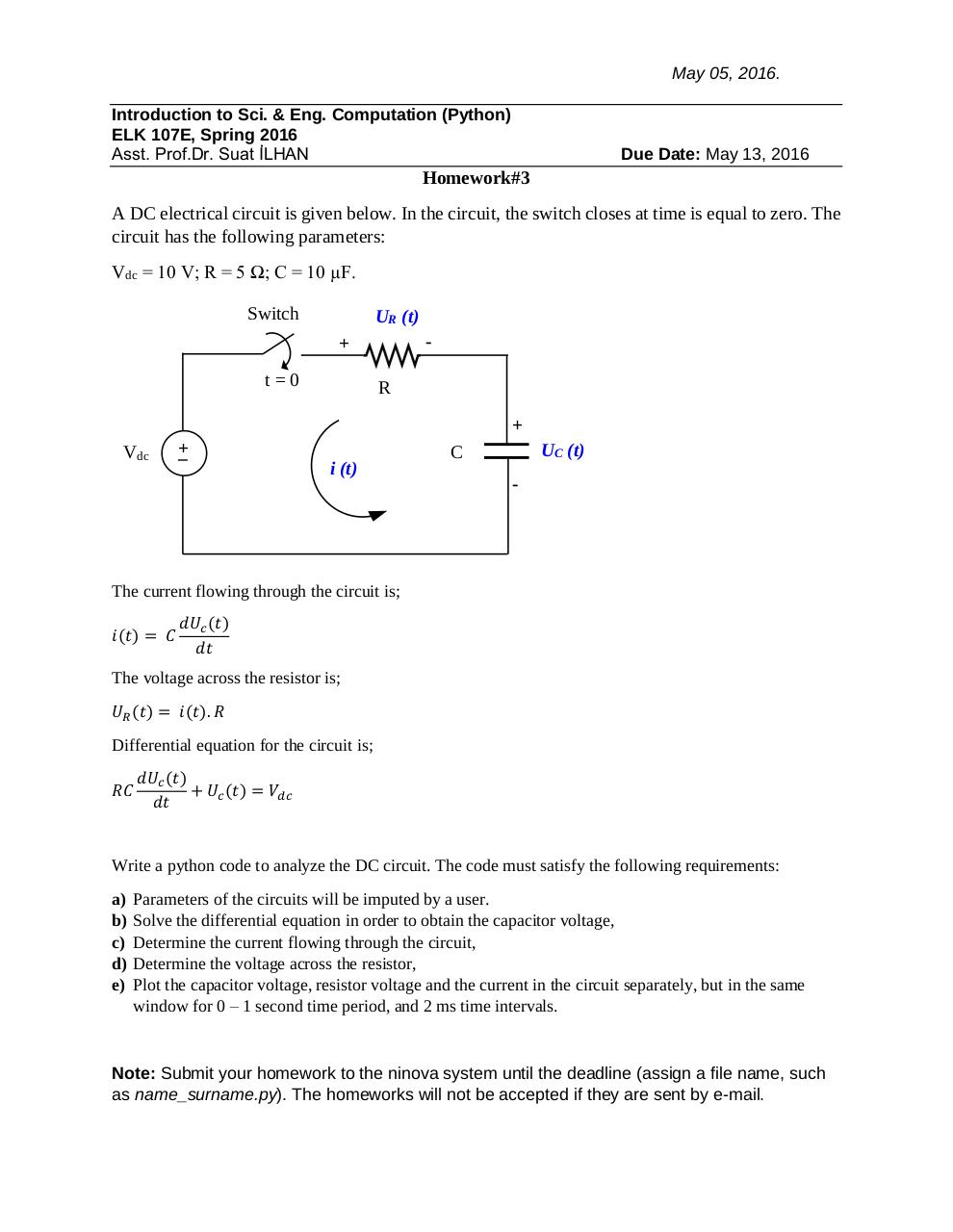# ELK107EHW3 .pdf

### File information

Original filename: ELK107EHW3.pdf
Author: suat

This PDF 1.5 document has been generated by MicrosoftÂ® Word 2013, and has been sent on pdf-archive.com on 12/05/2016 at 12:51, from IP address 161.9.x.x. The current document download page has been viewed 335 times.
File size: 325 KB (1 page).
Privacy: public file

ELK107EHW3.pdf (PDF, 325 KB)

### Document preview

May 05, 2016.
Introduction to Sci. &amp; Eng. Computation (Python)
ELK 107E, Spring 2016
Asst. Prof.Dr. Suat İLHAN

Due Date: May 13, 2016

Homework#3
A DC electrical circuit is given below. In the circuit, the switch closes at time is equal to zero. The
circuit has the following parameters:
Vdc = 10 V; R = 5 Ω; C = 10 µF.
Switch

UR (t)
-

+
t=0

R
+

Vdc

+
_
-

i (t)

UC (t)

C
-

The current flowing through the circuit is;
𝑖(𝑡) = 𝐶

𝑑𝑈𝑐 (𝑡)
𝑑𝑡

The voltage across the resistor is;
𝑈𝑅 (𝑡) = 𝑖(𝑡). 𝑅
Differential equation for the circuit is;
𝑅𝐶

𝑑𝑈𝑐 (𝑡)
+ 𝑈𝑐 (𝑡) = 𝑉𝑑𝑐
𝑑𝑡

Write a python code to analyze the DC circuit. The code must satisfy the following requirements:
a)
b)
c)
d)
e)

Parameters of the circuits will be imputed by a user.
Solve the differential equation in order to obtain the capacitor voltage,
Determine the current flowing through the circuit,
Determine the voltage across the resistor,
Plot the capacitor voltage, resistor voltage and the current in the circuit separately, but in the same
window for 0 – 1 second time period, and 2 ms time intervals.

Note: Submit your homework to the ninova system until the deadline (assign a file name, such
as name_surname.py). The homeworks will not be accepted if they are sent by e-mail.#### HTML Code

Copy the following HTML code to share your document on a Website or Blog

#### QR Code### Related keywords